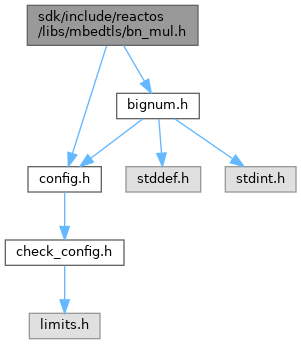ReactOS  0.4.15-dev-1184-g23e04ae
bn_mul.h File Reference

Multi-precision integer library. More...

`#include "config.h"`
`#include "bignum.h"`
Include dependency graph for bn_mul.h:Go to the source code of this file.

## Macros

#define asm   __asm

## Detailed Description

Multi-precision integer library.

Definition in file bn_mul.h.

## ◆ asm

 #define asm   __asm

Definition at line 79 of file bn_mul.h.

Value:
r = *(s++) * (mbedtls_t_udbl) b; \
r0 = (mbedtls_mpi_uint) r; \
r1 = (mbedtls_mpi_uint)( r >> biL ); \
r0 += c; r1 += (r0 < c); \
r0 += *d; r1 += (r0 < *d); \
c = r1; *(d++) = r0;
GLdouble GLdouble GLdouble r
Definition: gl.h:2055
static DNS_RECORDW r1
Definition: record.c:37
uint64_t mbedtls_t_udbl
Definition: bignum.h:195
#define b
Definition: ke_i.h:79
#define d
Definition: ke_i.h:81
uint32_t mbedtls_mpi_uint
Definition: bignum.h:193
GLdouble s
Definition: gl.h:2039
#define c
Definition: ke_i.h:80

Definition at line 887 of file bn_mul.h.

Value:
{ \
mbedtls_t_udbl r; \
mbedtls_mpi_uint r0, r1;
GLdouble GLdouble GLdouble r
Definition: gl.h:2055
static DNS_RECORDW r1
Definition: record.c:37

Definition at line 882 of file bn_mul.h.